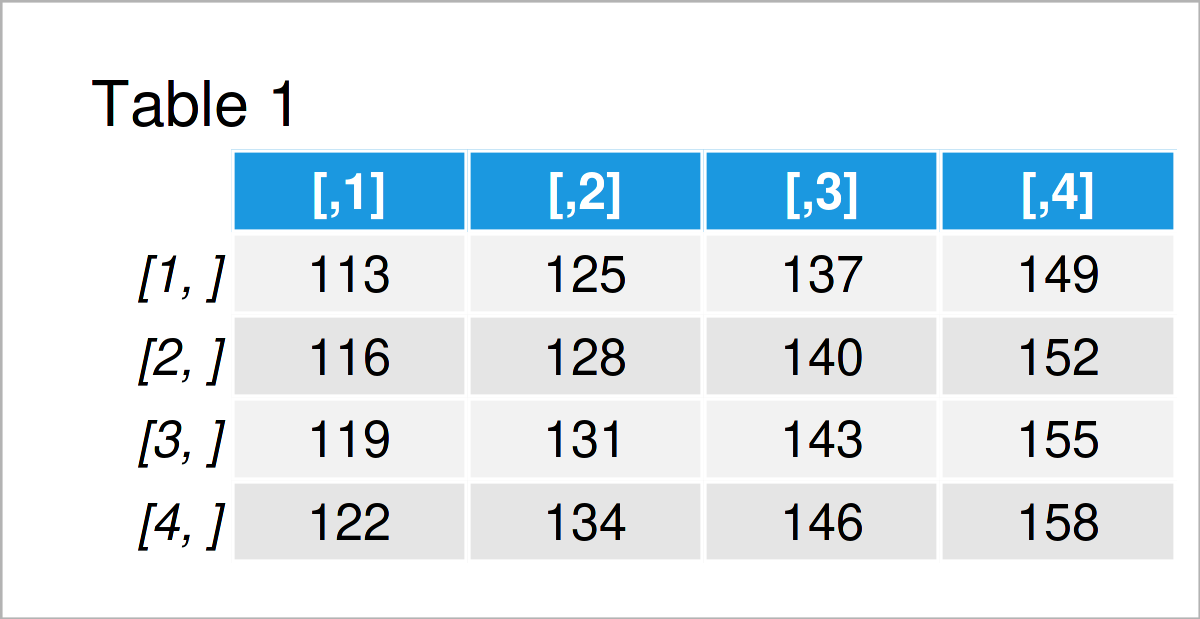# Sum a List of Matrices in R (Example)

In this article, I’ll show how to calculate the sum of all matrix objects in a list in the R programming language.

The tutorial contains these contents:

Let’s dive into it!

## Creation of Example Data

Have a look at the following example data:

```my_list <- list(mat1 = matrix(1:16, ncol = 4),    # Create example list of matrices
mat2 = matrix(11:26, ncol = 4),
mat3 = matrix(101:116, ncol = 4))
my_list                                           # Print example list of matrices
# \$mat1
#      [,1] [,2] [,3] [,4]
# [1,]    1    5    9   13
# [2,]    2    6   10   14
# [3,]    3    7   11   15
# [4,]    4    8   12   16
#
# \$mat2
#      [,1] [,2] [,3] [,4]
# [1,]   11   15   19   23
# [2,]   12   16   20   24
# [3,]   13   17   21   25
# [4,]   14   18   22   26
#
# \$mat3
#      [,1] [,2] [,3] [,4]
# [1,]  101  105  109  113
# [2,]  102  106  110  114
# [3,]  103  107  111  115
# [4,]  104  108  112  116```

The previous RStudio console output shows that our example data is a list object containing three different matrices.

## Example: Get Sum of All Matrices in List Using Reduce() Function

The following R code explains how to add the values in multiple matrices in a list.

For this task, we can apply the Reduce function as shown below:

```list_sum <- Reduce("+", my_list)                  # Calculate sum of matrices
list_sum                                          # Print sum of matrices```Have a look at the previous table: It shows the sum of the three matrices in our example list stored in a new matrix object called list_sum.

## Video & Further Resources

In case you need further information on the R programming codes of this tutorial, I recommend having a look at the following video on my YouTube channel. In the video, I’m explaining the topics of this tutorial.

Please accept YouTube cookies to play this video. By accepting you will be accessing content from YouTube, a service provided by an external third party.If you accept this notice, your choice will be saved and the page will refresh.

Furthermore, you might want to read the other articles on this website.

To summarize: You have learned in this article how to compute the sum of all matrices in a list in the R programming language. If you have additional questions, please let me know in the comments section.

Subscribe to the Statistics Globe Newsletter